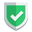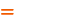我们相信：世界是美好的，你是我也是。平行空间的世界里面，不同版本的生活也在继续...

## 前文提示

`ndarray`之所以会给苏南大叔造成重大的意外，就是因为上文所说的两个对成员进行设置的地方。那么，本文也是基于上述的意外点展开阐述的。

## 初始值对后续值的影响不同

``````import numpy as np

s1 = [["苏", "南", "大", "叔"], ["北", "京", "大", "学"]]
s2 = np.array(s1)

s1[0:2]= "豪豪豪"
s2[0:2]= "豪豪豪"

print(s1)
print(s2)``````

``````[['苏', '南', '豪豪豪', '叔'], ['北', '京', '大', '学']]
[['苏' '南' '豪' '叔']
['北' '京' '大' '学']]``````

## 对一组数据设置的结果不同

``````import numpy as np
s1 = [["苏", "南", "大", "叔"], ["北", "京", "大", "学"]]
s2 = np.array(s1)

s1[0:1] = "叔"
s2[0:1] = "叔"

print(s1)
print(s2)``````

``````['叔', ['北', '京', '大', '学']]
[['叔' '叔' '叔' '叔']
['北' '京' '大' '学']]``````

``````import numpy as np
s1 = [["苏", "南", "大", "叔"], ["北", "京", "大", "学"]]
s2 = np.array(s1)

s1[:] = "叔"
s2[:] = "叔"

print(s1)
print(s2)``````

``````['叔']
[['叔' '叔' '叔' '叔']
['叔' '叔' '叔' '叔']]``````

## 输出不同

• `list`是用逗号隔开的，`[]`之间没有换行。
• `ndarray`是用空格隔开的，`[]`之间，多一层多个换行。
• `pycharm`可以对`ndarray`可视化，确不支持对`list`类型的可视化。

## 整体直接运算

• `list`是直接成员数量加倍。
• `ndarray`是数字类型数字翻倍，字符类型直接出错。
``````import numpy as np

s1 = [1,2]
s2 = np.array(s1)
print(s1*2)      # [1, 2, 1, 2]
print(s2*2)      # [2 4]

s1 = [[1,2]]
s2 = np.array(s1)
print(s1*2)      # [[1, 2], [1, 2]]
print(s2*2)      # [[2 4]]``````

``````import numpy as np

s1 = [[1,2], ["北", "京"]]
s2 = np.array(s1)
print(s1*2)      # [[1, 2], ['北', '京'], [1, 2], ['北', '京']]
print(s2*2)      # numpy.core._exceptions.UFuncTypeError: ufunc 'multiply' did not``````

``````Traceback (most recent call last):
File "C:\Users\sunan\Desktop\demo\py\t.py", line 15, in <module>
print(s2*2)
numpy.core._exceptions.UFuncTypeError: ufunc 'multiply' did not contain a loop with signature matching types (dtype('<U11'), dtype('<U11')) -> dtype('<U11')``````

## 结束语如果本文对您有帮助，或者节约了您的时间，欢迎打赏瓶饮料，建立下友谊关系。本博客不欢迎：各种镜像采集行为。请尊重原创文章内容，转载请保留作者链接。【福利】 腾讯云最新爆款活动！1核2G云服务器首年50元！【源码】本文代码片段及相关软件，请点此获取更多信息【绝密】秘籍文章入口，仅传授于有缘之人python